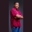Related Tags

character
c#
communitycreator

# How can we get the numeric value of a character in C#Theodore Kelechukwu Onyejiaku

### Overview

Some characters in C# are numeric Unicode. We can get the numeric values of these characters by using the Char.GetNumericValue() method. Numeric Unicode characters are present in strings. These characters are the numbers we know. Some examples of these characters are 1, 2, 3, and so on. These numbers are referred to as characters when they are used inside strings.

When we create a string that has numeric Unicode character(s), we can get the numeric value of any of those characters by using the Char.GetNumericValue() method and specifying the index position of the character.

### Syntax

public static double GetNumericValue (string s, int index);


### Parameters

s: This is the string that calls this method.

index: This is the index position of the character, in the string, whose numeric value we want to get.

### Return value

The value returned is a double value.

### Code example

In the code below, we will create some strings and get the numeric values of some of their characters, using the Char.GetNumericValue() method.

Note: If the character at position index is not a numeric character, -1 is returned.

using System;

// create class
class CharNumFinder
{
// create main method
static void Main()
{
// create strings
string s1 = "Edpresso";
string s2 = "id is 89";
string s3 = "1234";

// get numeric values of
// some characters
double a = Char.GetNumericValue(s1, 4);
double b = Char.GetNumericValue(s2, 6);
double c = Char.GetNumericValue(s3, 2);

// print out returned values
Console.WriteLine(a);
Console.WriteLine(b);
Console.WriteLine(c);
}
}
Getting the numeric value of a character in C#

RELATED TAGS

character
c#
communitycreator

CONTRIBUTORTheodore Kelechukwu Onyejiaku
RELATED COURSES

View all Courses

Keep Exploring

Learn in-demand tech skills in half the time